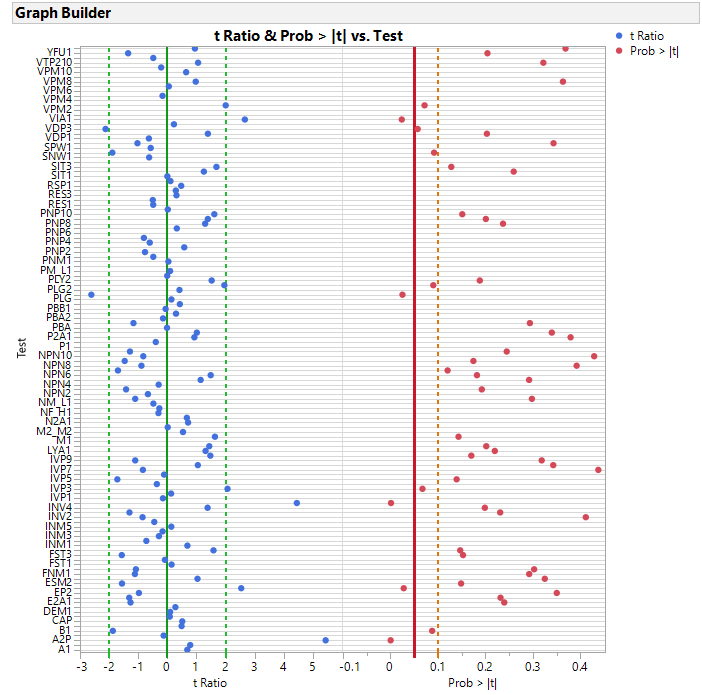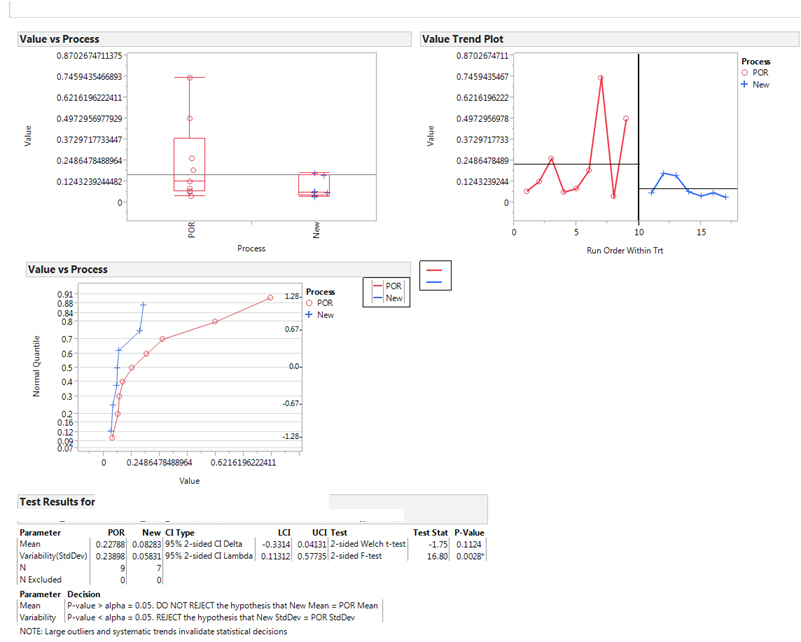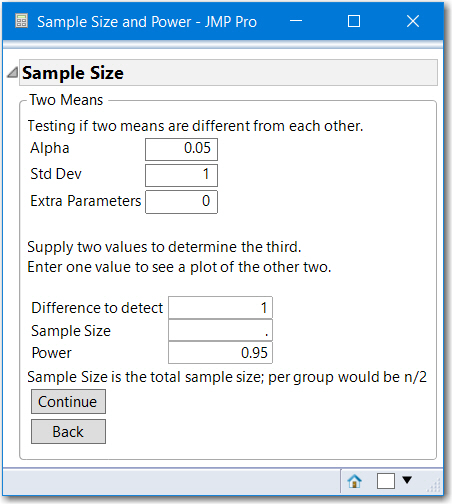Choose Language Hide Translation Bar
Highlighted

## How to show on graph only failed T test

Hi all,

i am using the following script in order to perform T test for between two groups (stressed abd control).

``````	Oneway(
Y( :Shift ),
X( :Group ),
by(:Test),
t Test( 1 ),
Box Plots( 1 ),
X Axis Proportional( 0 ),
Points Jittered( 1 ),
Grand Mean( 0 ),
SendToReport(
Dispatch(
{},
"Oneway Plot",
FrameBox,
{DispatchSeg(
Box Plot Seg( 1 ),
{Box Type( "Outlier" ), Line Color( "Red" )}
), DispatchSeg(
Box Plot Seg( 2 ),
{Box Type( "Outlier" ), Line Color( "Red" )}
)}
)
)
);``````

as you can see i am performing the T test for each "Test" and i have Thousands  of tests. my question if there is a option to show on the graph only the test where the T test got failed?

Thanks!!

1 ACCEPTED SOLUTION

Accepted Solutions
Highlighted

## Re: How to show on graph only failed T test

There are multiple ways to solve this question in JMP.  I prefer to use Response Screening to do a very fast run through of the data to get just the p-values of the analyses, and then take those results and select the desired data for analysis.  Below is a simple script that does that on some sample data I create to match your specific data table column names.

``````names default to here(1);

// Modify a sample data table to mimic your t-test code
dtSemi = open("\$SAMPLE_DATA/semiconductor capability.jmp", invisible);

dtSemi << select where( :Site > 2 );
dtSemi << delete rows;
dtSemi:Site << set name( "Group" );
colNames = dtSemi << get column names( continuous );

dt = dtSemi << stack( columns(colNames) ,
Source Label Column( "Test" ),
Stacked Data Column( "Shift" ),
Name( "Non-stacked columns" )(Keep( :Group ))
);

close( dtSemi, nosave );

// The above code just creates a sample data table....The code below generates
// the desired output

// Now run the Response Screening to get the failed t-tests
rs = dt << Response Screening( Y( :Shift ), X( :Group ), Grouping( :Test ) );
dtRS = rs << Get PValues;
//dtRS << show window( 0 );
report(rs) << close window;

// select all of the failed t-tests and get their test names
// We are using failue of .1 as the target.....just change the
// target value if you want .05 or something else as the failure value
dtRS << select where( :PValue <= .1 );

If( N Rows(dtRS << get selected rows ) > 0,
dtRS << delete rows;
);

failingTests = dtRS:Test << get values;

// close the dtRS data table that is no longer needed
close( dtRS, nosave );

// Now select the Tests in the original data table that are not significant
dt << select where( contains( failingTests, :Test ) );

If( N Rows( dt << get selected rows ) > 0,
dt << invert row selection;
dt << hide and exclude ;
dt << clear select;
);

Oneway(
Y( :Shift ),
X( :Group ),
by(:Test),
t Test( 1 ),
Box Plots( 1 ),
X Axis Proportional( 0 ),
Points Jittered( 1 ),
Grand Mean( 0 ),
SendToReport(
Dispatch(
{},
"Oneway Plot",
FrameBox,
{DispatchSeg(
Box Plot Seg( 1 ),
{Box Type( "Outlier" ), Line Color( "Red" )}
), DispatchSeg(
Box Plot Seg( 2 ),
{Box Type( "Outlier" ), Line Color( "Red" )}
)}
)
)
);
``````
Jim
6 REPLIES 6
Highlighted

## Re: How to show on graph only failed T test

There are multiple ways to solve this question in JMP.  I prefer to use Response Screening to do a very fast run through of the data to get just the p-values of the analyses, and then take those results and select the desired data for analysis.  Below is a simple script that does that on some sample data I create to match your specific data table column names.

``````names default to here(1);

// Modify a sample data table to mimic your t-test code
dtSemi = open("\$SAMPLE_DATA/semiconductor capability.jmp", invisible);

dtSemi << select where( :Site > 2 );
dtSemi << delete rows;
dtSemi:Site << set name( "Group" );
colNames = dtSemi << get column names( continuous );

dt = dtSemi << stack( columns(colNames) ,
Source Label Column( "Test" ),
Stacked Data Column( "Shift" ),
Name( "Non-stacked columns" )(Keep( :Group ))
);

close( dtSemi, nosave );

// The above code just creates a sample data table....The code below generates
// the desired output

// Now run the Response Screening to get the failed t-tests
rs = dt << Response Screening( Y( :Shift ), X( :Group ), Grouping( :Test ) );
dtRS = rs << Get PValues;
//dtRS << show window( 0 );
report(rs) << close window;

// select all of the failed t-tests and get their test names
// We are using failue of .1 as the target.....just change the
// target value if you want .05 or something else as the failure value
dtRS << select where( :PValue <= .1 );

If( N Rows(dtRS << get selected rows ) > 0,
dtRS << delete rows;
);

failingTests = dtRS:Test << get values;

// close the dtRS data table that is no longer needed
close( dtRS, nosave );

// Now select the Tests in the original data table that are not significant
dt << select where( contains( failingTests, :Test ) );

If( N Rows( dt << get selected rows ) > 0,
dt << invert row selection;
dt << hide and exclude ;
dt << clear select;
);

Oneway(
Y( :Shift ),
X( :Group ),
by(:Test),
t Test( 1 ),
Box Plots( 1 ),
X Axis Proportional( 0 ),
Points Jittered( 1 ),
Grand Mean( 0 ),
SendToReport(
Dispatch(
{},
"Oneway Plot",
FrameBox,
{DispatchSeg(
Box Plot Seg( 1 ),
{Box Type( "Outlier" ), Line Color( "Red" )}
), DispatchSeg(
Box Plot Seg( 2 ),
{Box Type( "Outlier" ), Line Color( "Red" )}
)}
)
)
);
``````
Jim
Highlighted

## Re: How to show on graph only failed T test

@txnelson (Jim), It is so funny, I also started a reply using a table made from Semiconductor Capability.jmp, made from lot summmaries of wafer summaries and randomly choosing lots to be in Group A or Group B. I saw your reply, so I will not write up the details. Attached is the data table and the script to produce the graph below, for say every 100 tests, or some logical grouping of tests.

In practice, it is good to add the F-test and p-value to compare std deviations and a comparison on n, the number of units tested for each group. Since @Adir52's groups were control and stressed, stressing could produce unmeasurable (empty) results.  Also, if there are thousands of tests, maybe like semiconductor tests, some tests could be correlated, say all PNP tests shifted up, but not all were significant. That would be displayed as t-ratios  greater than 0.Being a statistician, I must warn about statistical significance. Note for this randomly grouped data, 118 t-tests, (128 tests 10 had zero stdev ,no t-ratio), 11 were flagged with a p-value of < 0.10, which is the expected error rate  (and 5 tests with p-value < 0.05).  So there are many caveats with basing judgement on just p-values and just the test of the means.

Final caveat to @Adir52, if the measurements are reported by automated test equipment, order can be important.  If there is a time element,  I recommend something like this display for each test.Highlighted

## Re: How to show on graph only failed T test

do you have recommendation regarding to sample size of the control units.

the streesed units group going to be 80 units.

Highlighted

## Re: How to show on graph only failed T test

@Adir52, if possible it is recommended to have the same number of units in each group for a two sample comparison.

Sample size is the factor used to reduce the risk of a type II error.

"In statistical hypothesis testing, a type I error is the rejection of a true null hypothesis (also known as a "false positive" finding), while a type II error is the failure to reject a false null hypothesis (also known as a "false negative" finding). More simply stated, a type I error is to falsely infer the existence of something that is not there (confirming to common belief with false information), while a type II error is to falsely infer the absence of something that is present (going against the common belief with false information)."

Excerpt from https://en.wikipedia.org/wiki/Type_I_and_type_II_errors. Even though you state your null hypothesis is that the control and unstressed are different, the two-sample test (ANOVA for 2 groups) null hypothesis is that the means of Group 1 and Group 2 are equivalent, equal.  So you need to design your experiment so that the test has the power to detect a specific difference. For example, if you want to be 95% confident that your experiment will detect a 0.5 sigma difference, you can use JMP to help you selecet the number of units.

From the JMP main menu, select DOE and depending upon your version of JMP the menu or one of the submenus contains a Sample Size and Power calculator. In JMP 14, you will find it  by selecting DOE > Design Diagnostics. Then select Two Sample Means. Below is a screen shot. Since each test likley has a different standard deviation, this design step will be done with relative values. Specify Std Dev as 1 and then the Difference as the difference to detect. Below it is specified as 1. If you press Continue, the sample size will be 54 or 27 units for each group. Delete the sample size and specify the Difference to be 0.5 or one-half Std Dev, then press Continue, the Sample Size will be 210 or 105 units for each group. Or, delete Difference and enter 160 in for Sample Size (80 units each group), then Difference is reported as 0.57 or your test will have confidence that a difference of 0.57 sigma will be detected 95% of the time.If you have a statistician available or a university statistics professor near by familiar with your industry, I suggest you talk it over.  Your specific study could have a long history of the control, and the number of control units are just a check that the control is within the baseline.  But even more important, is the selection of the units, and how the experiment is run to make this a fair comparison and what population these conclusions apply.

Highlighted

## Re: How to show on graph only failed T test

Hi Txnelson
first of all thanks a lot for your input, its was very helpfull.
i had a wrong question. i want to display the tests where the Groups are not statistically equal. its easy using your code and delete the following line:
dt << invert row selection;

could you advise the meaning of the PValue?
is it = Prob>|t|?
i am asking this question because my H0 is that Group1=Group2
Highlighted

## Re: How to show on graph only failed T test

pValue is the probablility of your statistical test being significant.  Significance is historically defined as 1 in 20 (pValue <= .05) or 2 in 20 (pValue <= .1 ).  And yes, in your case, since your Ho is Group1=Group2, you would want to find where the pValue is > .05 or > .1.

Jim
Article Labels

There are no labels assigned to this post.